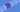# Python - If Statements

### 2020-04-19

Another one of the main uses of programming statements is to execute actions based on conditionals. In this article, we will take a breif look at if statements, and how you can use them.

Let's start with an example. Let's pretend we are writing a program for a movie theatre, and we want to very the price of ticket per a buyer's age. We can use if for this.

``````price = 18 # The standard price
age = 12 # The age of the buyer

if age < 18:
price = 10 # Discount if you are under 18

print(price)``````

Alright! Now, if the person is younger than 18, we change the variable price to \$12.

Now, let's introduce the **elif **statement to add a senior discount.

``````price = 18 # The standard price
age = 12 # The age of the buyer

if age < 18:
price = 10 # Discount if you are under 18
elif age > 65:
price = 8 # Discount if you are over 65

print(price)``````

Very nice. Now, if the person's age is greater than 65, we discount the price to \$8. Let's take a look at another way we can do the above.

``````price = 18 # The standard price
age = 12 # The age of the buyer

if age < 18:
price = 10 # Discount if you are under 18
if age > 65:
price = 8 # Discount if you are over 65

print(price)``````

Here, we get the same result, but both **if **statements will be checked. I wanted to show you that you don't always need the **elif. **There are also some advance reasons you will want to use this technique.

Finally, let's end by looking at the **else **statement.

``````price = 18 # The standard price
age = 12 # The age of the buyer

if age < 18:
price = 10 # Discount if you are under 18
elif age > 65:
price = 8 # Discount if you are over 65
else:
print("Sorry, no discount.")

print(price)``````

When our other conditions are not met, we can use the else statement. Here, we use the statement to tell users that they will not be receiving a discount.

And that's it for this lesson :D.

GoTea - KoalaTea